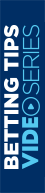Learn Why Should You Avoid Parlays

## Why Should You Avoid Parlays

It is a common opinion among expert bettors that to be successful at sports betting, you should avoid parlays. However, contrary to what the professionals think, parlay betting is prevalent. So, what is the reason for its popularity? It is simple–a parlay bet allows you to win much higher earnings than a simple bet, which attracts bettors. Sportsbooks encourage bettors to make these types of bets. But have you ever wondered why bookies invite punters to place parlay bets?

### High Odds And Minimal Hit Odds

Also called accumulators, parlays consist of unifying several simple bets in a single bet. Thanks to this, we can tailor a bet with high odds to suit us. We can calculate a two-match parlay's odds by multiplying the odds of each chosen event. Suppose we bet that two soccer games end in a draw. Also, let's imagine that the tie odds in each match would be 3.7 and 3.8. In that case, the odds of our parlay would be 3.7 x 3.8 = 14.06. As we can see, we will obtain a very high odd.

If we create, for example, a parlay with four single bets with odds of 1.5, 2.0, 4.0, and 2.5, respectively, the parlay odd will be 1.5 x 2.0 x 4.0 x 2.5 = 30. This is a very attractive odd, isn't it? However, we must observe that as the odds increase, our success probability decreases. We can calculate the odds' implicit probability as the odds' inverse. In our first example, the implicit probability was equal to 1 / 14.06 = 0.0711. That is, our winning probability is 7.11%

In the second example, the odds are 30, and our hitting probability is 3.33%. We can see our chances of winning drastically decrease by placing parlay bets. The risk we run is very high.

### Beware Of The Bookie's Spread When Placing Parlay Bets

All odds of a bookmaker carry a spread. This spread reduces the odds concerning the event's real occurring probability and represents the bookie's profit. There is a relatively simple formula to estimate this spread, and it is the following:

Bookie's spread = 1 - [(1 - simple spread) ^ Number of bets]

If we do a three-game parlay with an average spread of 5%, we will have the following:

Bookie's spread = 1 - [(1 - 0.05) ^ 3] = 1 - (0.95 x 0.95 x 0.95) = 0.143

So, this means that the bookie's commission is 14.3%. The spread almost tripled when combining three bets! Remember that the spread is the bookmaker's profit. So, the reason for the great interest of the bookies in which we place parlays is clear.

### Conclusion

After the above, it is clear that we will have very low hitting probabilities if we combine bets to obtain very high odds. In this way, our profits will be much lower. We could even fall into long losing streaks that lead us to lose our bankroll. We must also bear in mind the effect of the bookie spread. When we place an accumulator bet, we must place all bets with the same sportsbook. Therefore, we cannot benefit from reducing the spread by looking for the most convenient odds in different sportsbooks.

• Share:

Payment MethodJoin www.soccertipsters.com today
and start receiving our consistent soccer tips.

NEXT
Be The
Winner

Join www.soccertipsters.com today
and start receiving our consistent soccer tips.

Thank You
;
for subscribing to our newsletter

Stay tuned for more exclusive information from SoccerTipsters!

X
x Close
Today Tips
Tipster
soccertipsters
Customer support
Opportunity